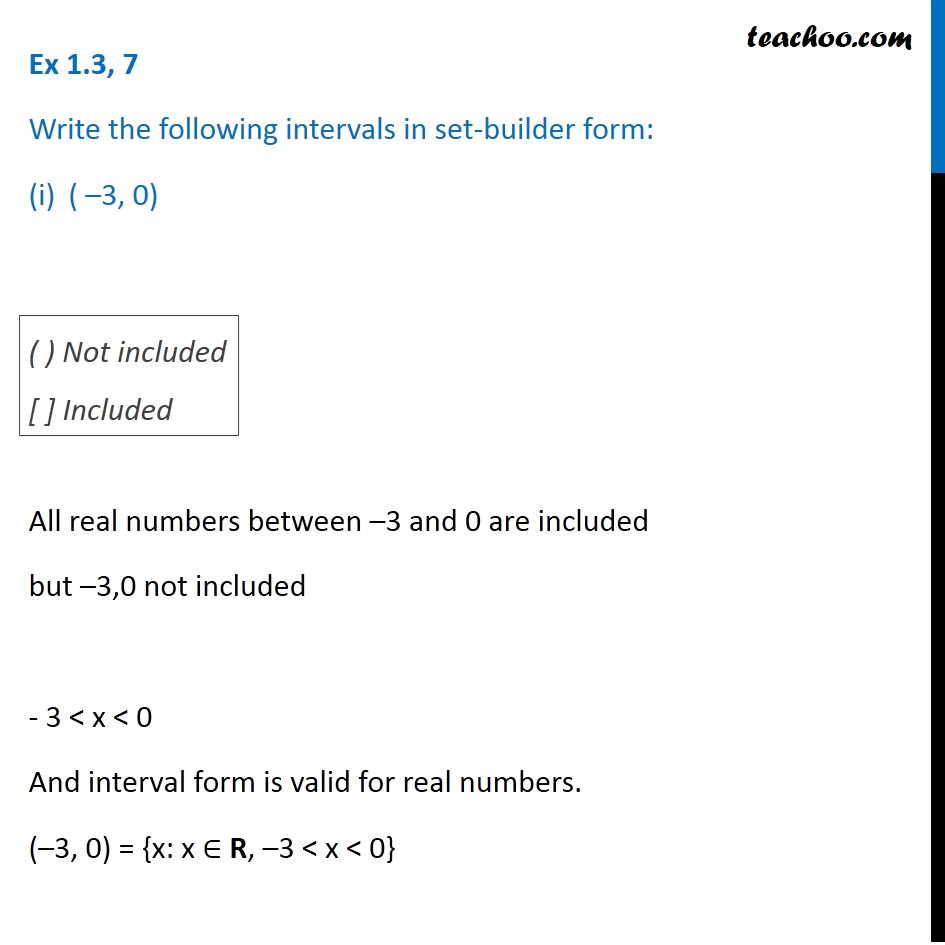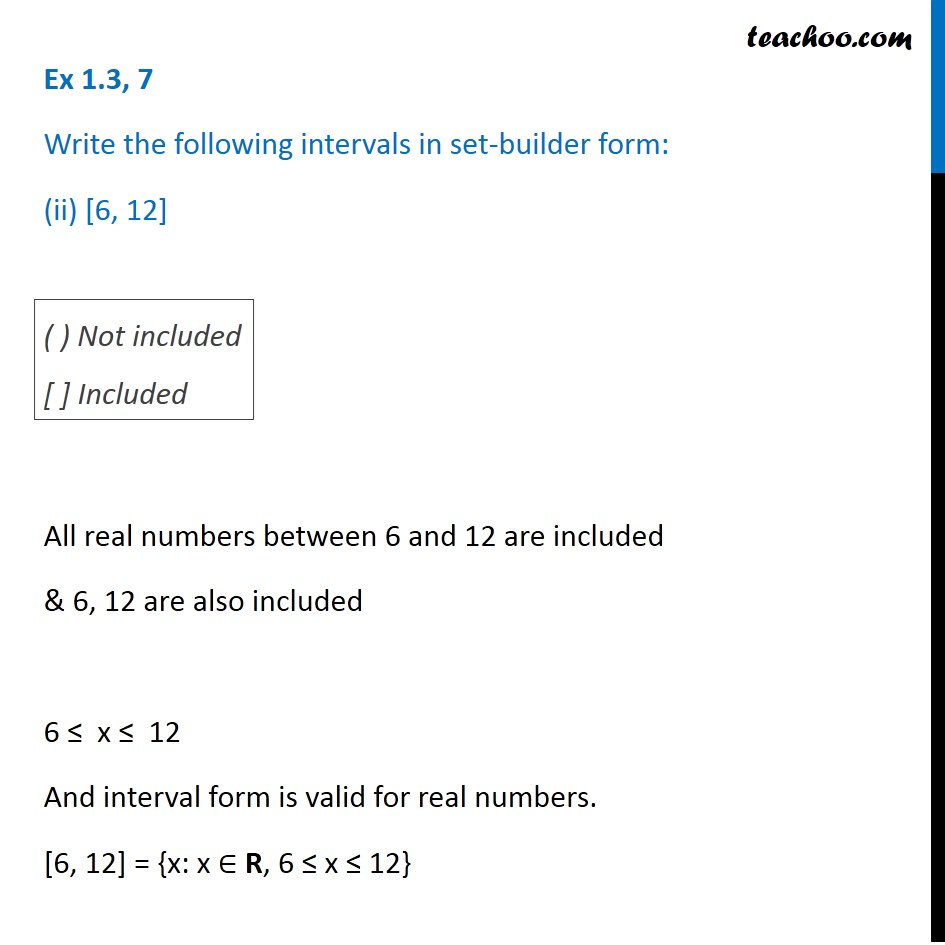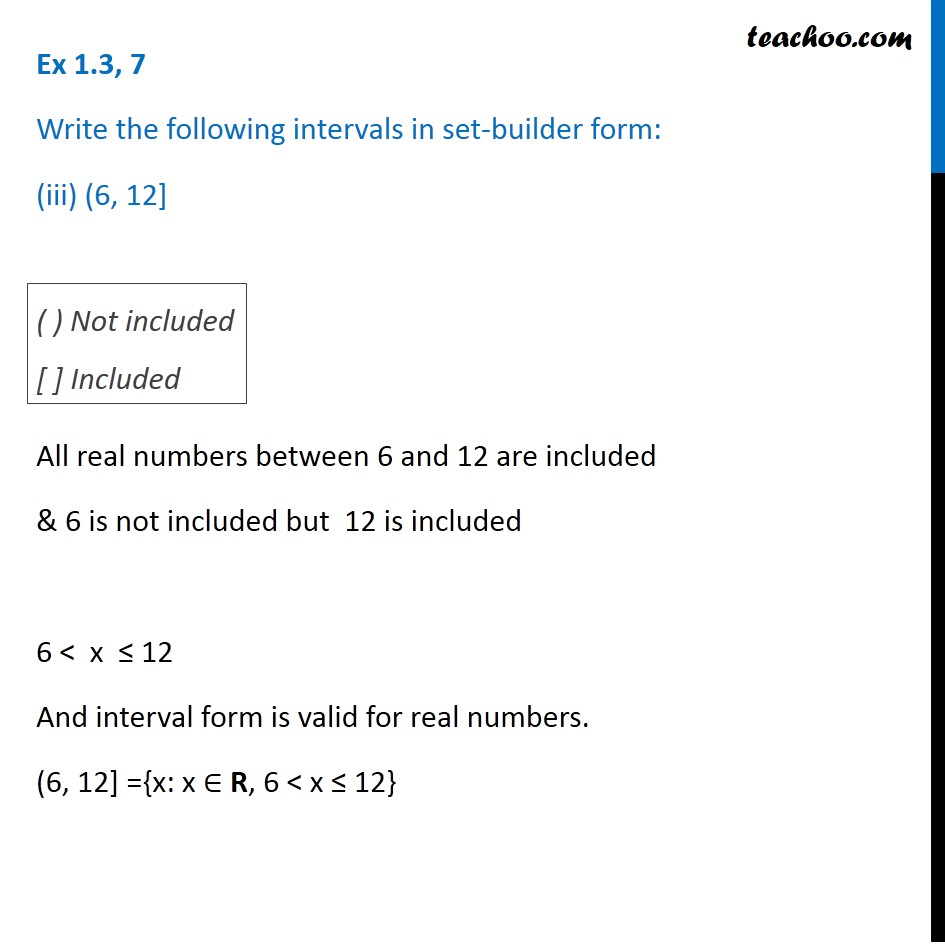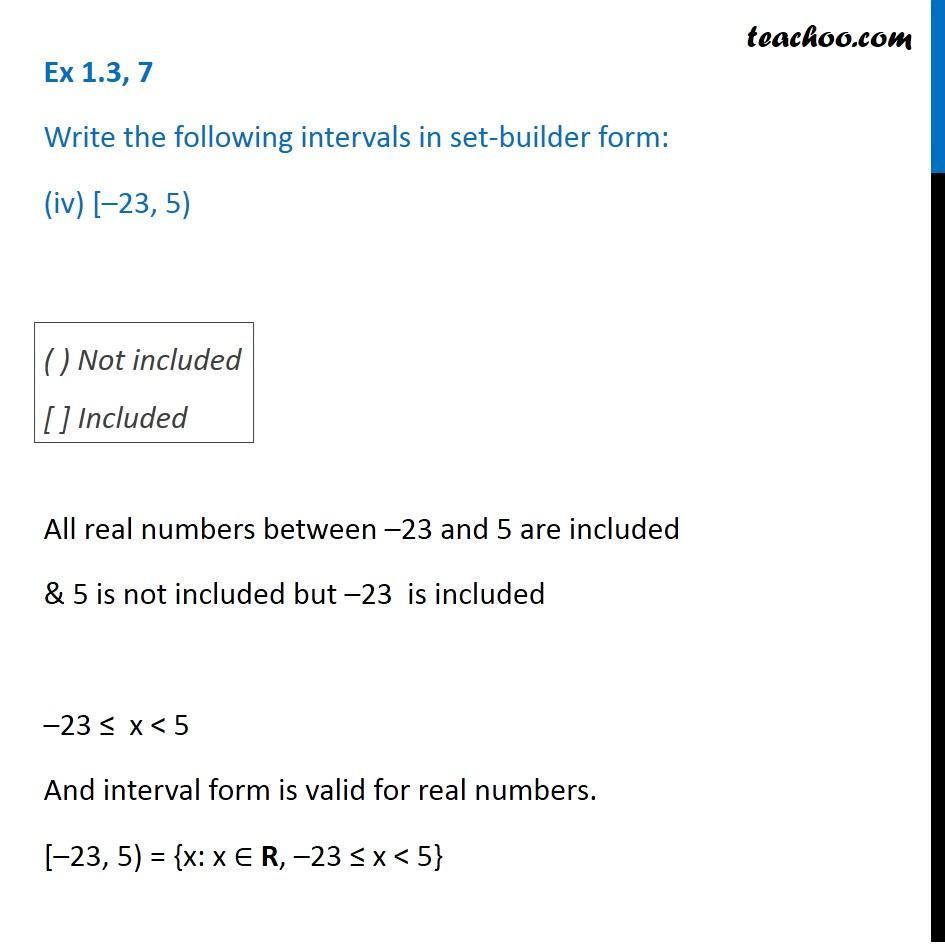1. Chapter 1 Class 11 Sets
2. Concept wise
3. Intervals

Transcript

Ex 1.3, 7 Write the following intervals in set-builder form: ( –3, 0) All real numbers between –3 and 0 are included but –3,0 not included - 3 < x < 0 And interval form is valid for real numbers. (–3, 0) = {x: x ∈ R, –3 < x < 0} ( ) Not included [ ] Included Ex 1.3, 7 Write the following intervals in set-builder form: (ii) [6, 12] All real numbers between 6 and 12 are included & 6, 12 are also included 6 ≤ x ≤ 12 And interval form is valid for real numbers. [6, 12] = {x: x ∈ R, 6 ≤ x ≤ 12} ( ) Not included [ ] Included Ex 1.3, 7 Write the following intervals in set-builder form: (iii) (6, 12] All real numbers between 6 and 12 are included & 6 is not included but 12 is included 6 < x ≤ 12 And interval form is valid for real numbers. (6, 12] ={x: x ∈ R, 6 < x ≤ 12} ( ) Not included [ ] Included Ex 1.3, 7 Write the following intervals in set-builder form: (iv) [–23, 5) All real numbers between –23 and 5 are included & 5 is not included but –23 is included –23 ≤ x < 5 And interval form is valid for real numbers. [–23, 5) = {x: x ∈ R, –23 ≤ x < 5} ( ) Not included [ ] Included

Intervals